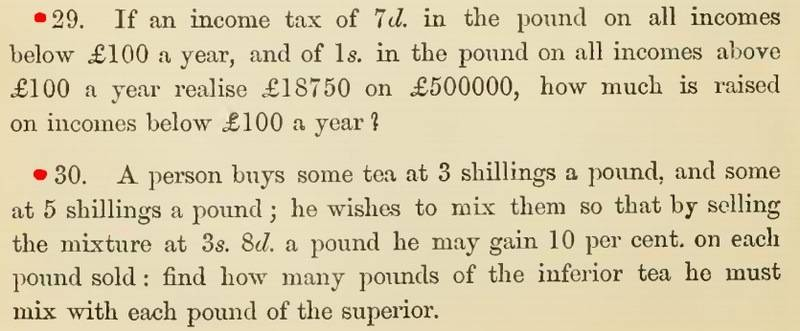# Word problem algebra

MENTOR NOTE: Moved here from the General Math forum hence no homework template.

Hello! Sorry for not showing what I have tried to solve the problem.

Here it is
I let x = amount of inferior tea to be added to superior tea in lbs.
y = amount of superior tea in lbs.
3x+5y = 11/3(x+y)
solving for x I get x = 2y from here I cannot proceed.
Honestly this is only where I can get to. Please bear with me.Last edited by a moderator:

jedishrfu
Mentor
First 3s 8d is 3.666 s = 11/3 shillings right?

There are two equations:

3x + 5y = 11/3

x + y = 1

Try subbing the second one into the first one.

First 3s 8d is 3.666 s = 11/3 shillings right?

There are two equations:

3x + 5y = 11/3

x + y = 1

Try subbing the second one into the first one.
Yes, 3s. 8d is 11/3 s.

Solving for x. x = 1-y and substituting it to equation 3x+5y=11/3
3-3y+5y=11/3.
Solving for y. y= 1/3
Solving for x=2/3 lbs? But the answer in my book is 5lbs? Why is that?

jedishrfu
Mentor
Ahh I didn't read the problem completely. There's a 10 percent feature too so the 11/3 shilling should be (11/3) = (110/100) * z ie z=10/3 shilling for the first equation.

From the two equations you will have the ratio of x pds inferior tea to y pds superior tea to make one pound mixed tea.

Then you have to ask yourself given 1 pd of superior tea how much inferior tea do I need to mix in to make the mixed tea.

Last edited:
Hello I get for y, y = 3/20
For x, x = 17/20

What do these quantities represent? Because in my post above x is defined as the required value. Im still confused
And why multiply 11/3 by 9/10?

jedishrfu
Mentor
I made a mistake you should use 10/3 not 11/3 as I didn't interpret the 10% feature correctly.

The cost of the mix is 10/3 and he wants to make a 10% profit ie 11/3 --> 10/3 * 11/10 = 11/3

This adjustment gives you better x and y values.

I get x = 5/6 and y=1/6

My question is, in the system of equation in your post above, what do x and y represent?

jedishrfu
Mentor
You can't tell from the equations?

Look at the first equation what are we adding together here?

If it is the same thing as what I defined them in my first post the answer is 5/6 lbs?

jedishrfu
Mentor
Yes and so now you should have the answer and it should agree with your book.

Hello! But the answer in my book is 5 lbs not 5/6 lbs? Sorry, english is not my primary language. That is why Im having a hard time analysing the problem.

jedishrfu
Mentor
x=5/6 pds and y=1/6 pds

x = ?? when y = 1 pd

•paulmdrdo
I get it now! Thank you so much!

•jedishrfu
jedishrfu
Mentor
I had trouble with the problem too though not the english but the shilling and pence money issue. We use dollars and cents. I learned that there 12 pence to a shilling.

•paulmdrdo
so it seems that the last question was about the mixture ratio?

typically mixture ratio is given as (5) : (1) or something similar. It's noted in integers.

1.weight is the same as the money (lbs = pound, and sterling= pound)
2.there are shillings and percentages.
3. I have to admit that the percentage profit got me confused as well.

the profited price looks like it is the price for the customer in the store. The price that the tea-mixer pays, is a different price, and should be the original price which we should use, I think... Tea-mixer ups the price, to get value for his work,time and effort (?). This way, he can stay in business and keep getting money.

1.1 * p = 11/3 shillings [price to customers 3 shillings and 8 pence]
p = (11/3) / 1.1
p= 10/3 [price that tea-mixer pays himself]

p should be lower value in magnitude compared to 11/3, because otherwise the business venture will go bankrupt.